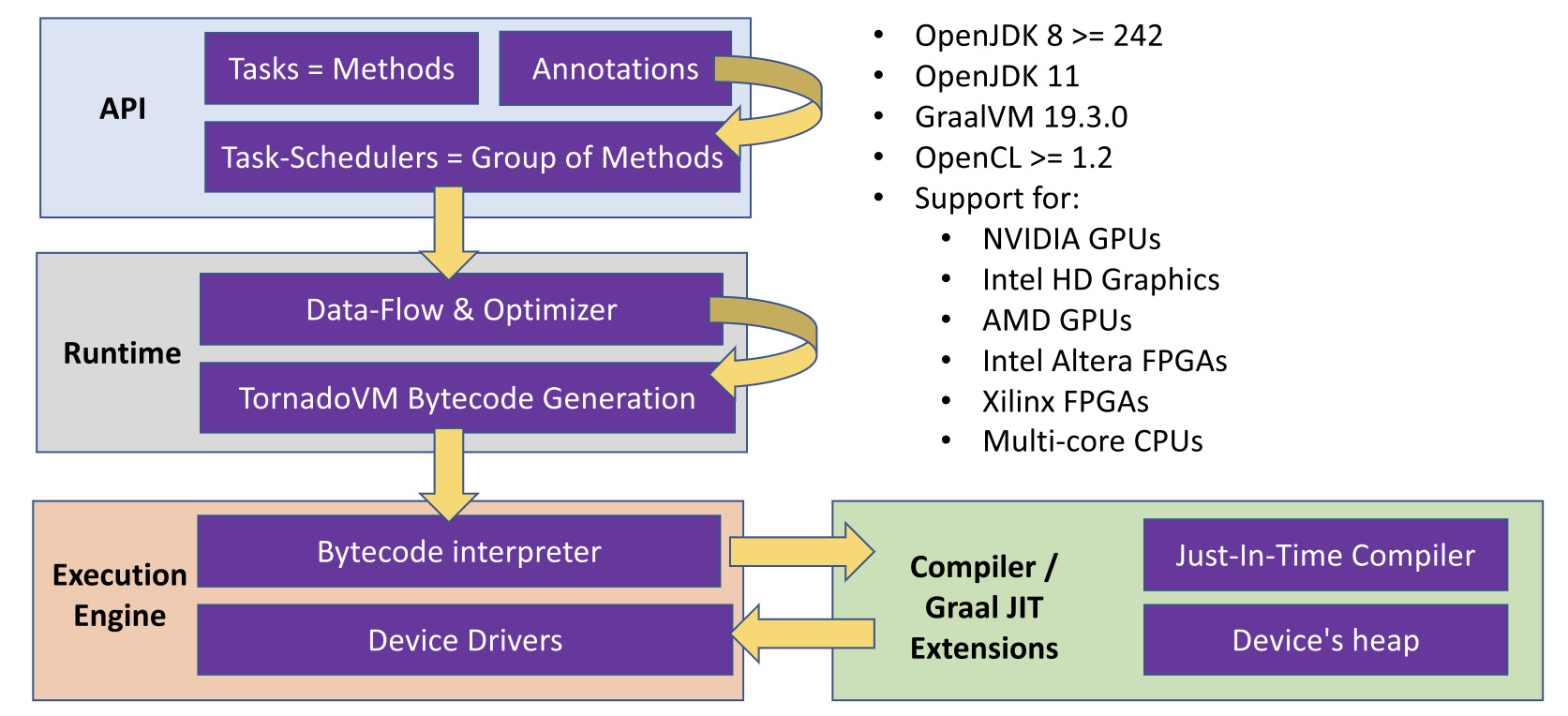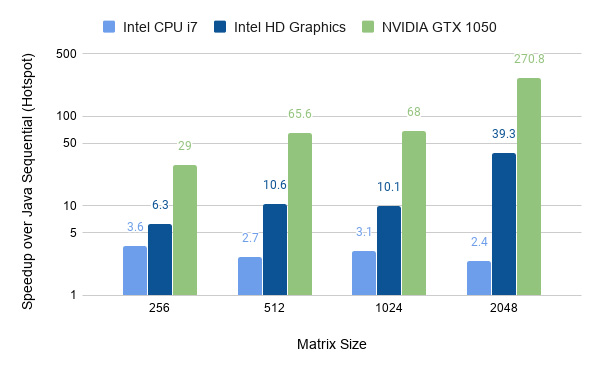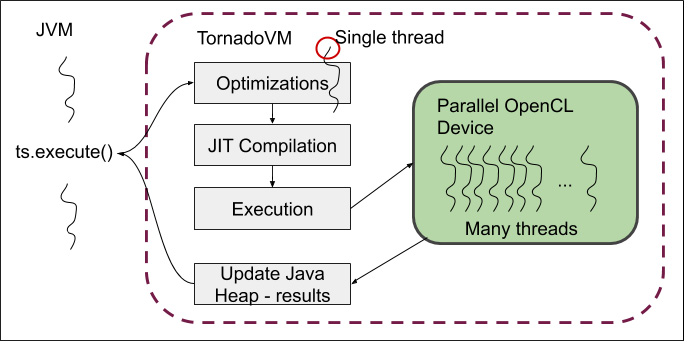fpga深度学习gpu加速

### 重要要点

OpenCL的目标是希望编写可移植但高效的代码的专业程序员。 [...]因此，OpenCL提供了底层硬件抽象以及支持编程的框架，并且公开了底层硬件的许多细节。

## 让我们开始吧！``````class Compute {
public static void matrixMultiplication(final float[] A, final float[] B, final float[] C, final int size) {
for (int i = 0; i < size; i++) {
for (int j = 0; j < size; j++) {
float sum = 0.0f;
for (int k = 0; k < size; k++)
sum += A[(i * size) + k] * B[(k * size) + j];
C[(i * size) + j] = sum;
}
}
}
}``````

``````class Compute {
public static void matrixMultiplication(final float[] A, final float[] B, final float[] C, final int size) {
for (@Parallel int i = 0; i < size; i++) {
for (@Parallel int j = 0; j < size; j++) {
float sum = 0.0f;
for (int k = 0; k < size; k++)
sum += A[(i * size) + k] * B[(k * size) + j];
C[(i * size) + j] = sum;
}
}
}
}``````

`@Parallel`批注由TornadoVM JIT编译器（将Java字节码转换为OpenCL）用作提示。

TornadoVM JIT编译器不会强制并行化。 相反，它检查带注释的循环是否可以并行化，并在OpenCL（ `get_global_id(dimension)``get_global_id(dimension)` for循环替换等效的并行索引。 如果不能并行处理for循环，则TornadoVM退出并执行顺序代码。

``````TaskSchedule t = new TaskSchedule("s0")
.task("t0", Compute::matrixMultiplication, matrixA, matrixB, result, size)
.streamOut(result);``````

### 运行矩阵乘法：设置TorandoVM

``````\$ mkdir -p TornadoVM
\$ tar -xf graalvm-ce-java11-linux-amd64-19.3.0.tar.gz
\$ export JAVA_HOME=\$PWD/graalvm-ce-java11-19.3.0
\$ git clone --depth 1 https://github.com/beehive-lab/TornadoVM
\$ export PATH=\$PWD/bin/bin:\$PATH
\$ export CMAKE_ROOT=<SET YOUR PATH TO CMAKE ROOT>
\$ make graal-jdk-11

``````\$ git clone https://github.com/jjfumero/qconlondon2020-tornadovm
\$ export JAVA_HOME=/path/to/graalvm-ce-java11-19.3.0
\$ export PATH="\${PATH}:\${TORNADO_ROOT}/bin/bin/"  ## Defined previously
\$ mvn clean install``````

``````\$ tornado --devices
Total number of devices  : 3

NVIDIA CUDA -- GeForce GTX 1050
Global Memory Size: 3.9 GB
Local Memory Size: 48.0 KB
Workgroup Dimensions: 3
Max WorkGroup Configuration: [1024, 1024, 64]
Device OpenCL C version: OpenCL C 1.2

Intel(R) OpenCL -- Intel(R) Core(TM) i7-7700HQ CPU @ 2.80GHz
Global Memory Size: 31.0 GB
Local Memory Size: 32.0 KB
Workgroup Dimensions: 3
Max WorkGroup Configuration: [8192, 8192, 8192]
Device OpenCL C version: OpenCL C 1.2
Intel(R) OpenCL HD Graphics -- Intel(R) Gen9 HD Graphics NEO
Global Memory Size: 24.8 GB
Local Memory Size: 64.0 KB
Workgroup Dimensions: 3
Max WorkGroup Configuration: [256, 256, 256]
Device OpenCL C version: OpenCL C 2.0``````

``\$ tornado qconlondon.MatrixMultiplication 512 tornado``

``````\$ tornado qconlondon.MatrixMultiplication 512 tornado
Computing MxM of 512x512
Total time: 77568790 (ns), 0.0776 (s)
Total time: 3133182 (ns), 0.0031 (s)
Total time: 3126146 (ns), 0.0031 (s)
…``````

``````\$ tornado --debug qconlondon.MatrixMultiplication 512 tornado
Computing MxM of 512x512
platform          : NVIDIA CUDA
device            : GeForce GTX 1050 CL_DEVICE_TYPE_GPU (available)
dims              : 2
global work offset: [0, 0]
global work size  : [512, 512]
local  work size  : [32, 32, 1]``````

``````\$ tornado qconlondon.MatrixMultiplication 512 sequential
Computing MxM of 512x512
Total time: 259398036 (ns), 0.2594 (s)
Total time: 247857535 (ns), 0.2479 (s)
...``````

### 如何更换设备？

``````\$ tornado -Ds0.t0.device=0:2 --debug qconlondon.MatrixMultiplication 512 tornado
Computing MxM of 512x512
platform          : Intel(R) OpenCL HD Graphics
device            : Intel(R) Gen9 HD Graphics NEO CL_DEVICE_TYPE_GPU (available)
dims              : 2
global work offset: [0, 0]
global work size  : [512, 512]
local  work size  : [16, 16, 1]``````

### 在Dell XPS 15笔记本电脑上运行的TornadoVM for MxM的性能## 执行模型与编译``````\$ tornado --printBytecodes qconlondon.MatrixMultiplication 512 tornado

vm: COPY_IN [F@3e694b3f on NVIDIA -- GeForce GTX 1050
vm: COPY_IN [F@397fbdb on NVIDIA -- GeForce GTX 1050
vm: COPY_IN [F@33d512c1 on NVIDIA -- GeForce GTX 1050
vm: LAUNCH task s0.t0-matrixMultiplication on NVIDIA -- GeForce GTX 1050
vm: STREAM_OUT_BLOCKING [F@33d512c1 on NVIDIA -- GeForce GTX 1050``````

## 分析OpenCL生成的代码

``\$ tornado --printKernel qconlondon.MatrixMultiplication 512 tornado``

TornadoVM在任务计划中为每个任务生成一个内核。 此外，它`lookupBufferAddress`生成一个名为`lookupBufferAddress`的内核，该内核将在VM引导期间执行。 该内核背后的原因是，TornadoVM仅分配一个大缓冲区来充当目标设备上的堆。 为此，它需要一个有效的指针，该指针将用作目标设备的基本地址，TornadoVM可以在该目标设备中执行数据传输。 `lookupBufferAddress`内核返回此基本指针。

``````__kernel void lookupBufferAddress(...parameters) {
__global ulong *_frame = (__global ulong *) &_heap_base[_frame_base];
_frame  =  (ulong) _heap_base;
}

__kernel void matrixMultiplication(...parameters) {
// Variables declaration …

__global ulong *_frame = (__global ulong *) &_heap_base[_frame_base];
// Access elements within the stack-frame
ul_0  =  (ulong) _frame;   // base address of input matrix A
ul_1  =  (ulong) _frame;   // base address of input matrix B
ul_2  =  (ulong) _frame;   // base address of input matrix C
i_3  =  get_global_id(1);     // Parallel OpenCL indexing (2nd dimension)
i_4  =  i_3;
for(;i_4 < 512;)  {
i_5  =  get_global_id(0);   // Parallel OpenCL indexing (1st dimension)
i_6  =  i_5;
for(;i_6 < 512;)    {
i_7  =  i_4 << 9;
f_8  =  0.0F;
i_9  =  0;
for(;i_9 < 512;)      {
i_10  =  i_9 + 1;
i_11  =  i_7 + i_9;
l_12  =  (long) i_11;
l_13  =  l_12 << 2;
l_14  =  l_13 + 24L;                  // Skip Java object header
ul_15  =  ul_0 + l_14;
f_16  =  *((__global float *) ul_15); // Load element from matrix A
i_17  =  i_9 << 9;
i_18  =  i_17 + i_6;
l_19  =  (long) i_18;
l_20  =  l_19 << 2;
l_21  =  l_20 + 24L;
ul_22  =  ul_1 + l_21;
f_23  =  *((__global float *) ul_22);// Load element from matrix B

f_24  =  fma(f_16, f_23, f_8);       // Computation (fuse-multiple-add)
f_8  =  f_24;
i_9  =  i_10;
}
i_25  =  i_6 + i_7;
l_26  =  (long) i_25;
l_27  =  l_26 << 2;
l_28  =  l_27 + 24L;
ul_29  =  ul_2 + l_28;
*((__global float *) ul_29)  =  f_8;    // Store the result in Matrix C
i_30  =  get_global_size(0);
i_31  =  i_30 + i_6;
i_6  =  i_31;
}
i_32  =  get_global_size(1);
i_33  =  i_32 + i_4;
i_4  =  i_33;
}
}``````

## 摘要